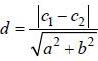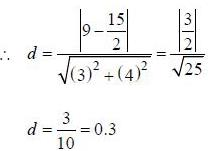Courses

# Mathematics MCQ 1

## 21 Questions MCQ Test Mock Test Series of IIT JAM Biotechnology | Mathematics MCQ 1

Description
This mock test of Mathematics MCQ 1 for IIT JAM helps you for every IIT JAM entrance exam. This contains 21 Multiple Choice Questions for IIT JAM Mathematics MCQ 1 (mcq) to study with solutions a complete question bank. The solved questions answers in this Mathematics MCQ 1 quiz give you a good mix of easy questions and tough questions. IIT JAM students definitely take this Mathematics MCQ 1 exercise for a better result in the exam. You can find other Mathematics MCQ 1 extra questions, long questions & short questions for IIT JAM on EduRev as well by searching above.
QUESTION: 1

### If Z is a complex number, then the minimum value of |z| + |z-l| is

Solution:

Note that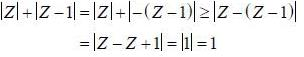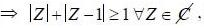, hence minimum value is 1 and it is attained at Z = 0, 1/2

QUESTION: 2

### The product of all real roots of the equation x2 - |x| - 6 = 0 is

Solution:

Equation is x2 - |x| - 6 = 0
Case I: x > 0. then we have
x2 - x - 6 = 0 (lx| = x)
⇒ (x-3)(x+2) = 0
⇒ x = 3 is the solution as x > 0.
(So x = -2 can’t be solution)
Case II: x < 0. then we have
x2 + x - 6 = 0    (|x| = -x)
⇒ (x + 3)(x - 2) = 0
⇒ x = -3 is the solution as x < 0
(So x = 2 can't be solution)
∴ product of roots = 3. - 3 = -9

QUESTION: 3

### The sum of the series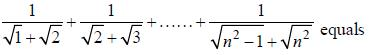Solution: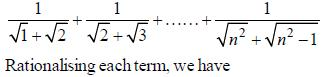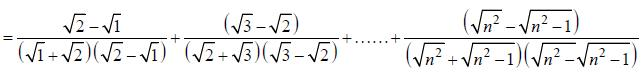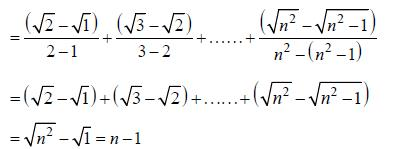QUESTION: 4

Two dice are rolled simultaneously. The probability that the sum of the two numbers on the top faces will be at least 10 is

Solution:

Two dice are rolled simultaneously, hence total number of elements in sample space is = 6 * 6 = 36
We have event E is the collection of those elements having sum greater or equal than 10.
i.e. E = {(4,6),(5.6).(6.6).(6.5).(6.4).(5.5)}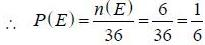QUESTION: 5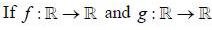are defined by f(x) = 2x + 3 and g (x) = x2 + 7, Then the value of x such that g (f(x)) = 8 are

Solution:

Given that f(x) = 2x + 3, g(x) = x2+7
∴   g(f(x)) = g(2x + 3) = (2x + 3)2 + 7
= 4x2 + 9 + 12x+ 7 = 4x2 + 12x + 16
Given that g (f (x)) = 8
⇒ 4x2 + 12x + 16 = 8
⇒ 4x2 + 12x + 8 = 0
⇒ 4(x2 + 3x + 2) = 0
⇒ 4(x + l)(x + 2) = 0
∴ x = -1 and x = -2

QUESTION: 6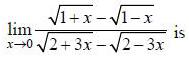Solution: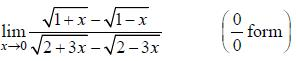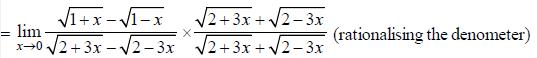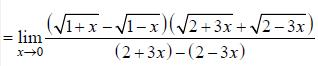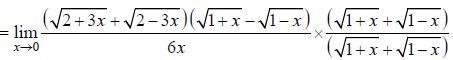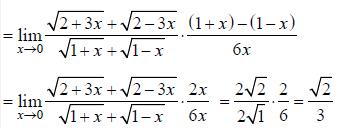Hence limit lies between 0 and 1/2

QUESTION: 7

The area bounded by the curves y = |x| -1 and y = - |x| +1 is

Solution: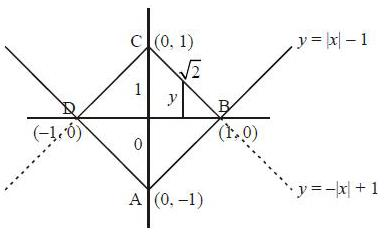Method-I: From the figure, it is clear that ABCD fonn a square having each side √2.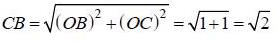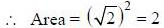Method -II: Area ofABCD = 4 x Area of OBC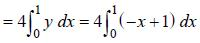(as equation of CB  is y = -x+ 1)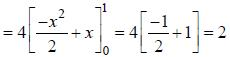QUESTION: 8

If X and 7are two sets, then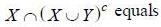Solution: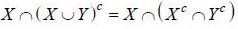(using De'morgans law)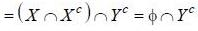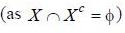= φ

QUESTION: 9

Let R = {(3,3),(6.6),(9,9),(12,12),(6.12),(3,9),(3,12),(3,6)} be a relation on the se A = {3,6,9,12}. The relation is

Solution:

(d) : For (3, 9) ∈ R, (9, 3) ∉ R

Therefore,relation is not symmetric which means our choice

(a) and (b) are out of court. We need to prove reflexivity and transitivity.

For reflexivity a ∈ R, (a, a) ∈ R which is hold i.e. R is reflexive. Again,

for transitivity of (a, b) ∈ R , (b, c) ∈ R

⇒ (a, c) ∈ R

which is also true in R = {(3, 3)(6, 6), (9, 9), (12, 12), (6,12), (3, 9), (3, 12), (3, 6)}.

QUESTION: 10

The radius of the circle x2 + y2 - 2x + 4y = 8

Solution:

Given circle x2 + y2 - 2x + 4y - 8 = 0
Comparing with the general equation of circle x2 + y2 + 2gx + 2fy - c = 0, we get g = -1. f = 2, c = -8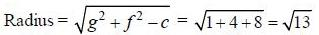*Multiple options can be correct
QUESTION: 11

In throwing a die, let
A be the event 'coining up of an odd number'
B be the event 'coming up of an even number'
C' be the event ‘coming up of a number > 4' and D be the event ‘coming up of a number <3'
Then

Solution:

For throwing a dice, sample space S = {1,2,3,4,5,6} and
A = {1,3,5}
B = {2,4,6}
C = {4,5,6}
D = {1,2}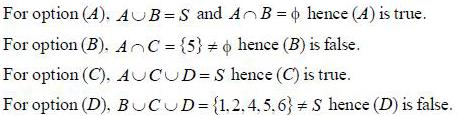*Multiple options can be correct
QUESTION: 12

The function f(x) = |x|+|x - 1| is

Solution:

Given that f (x) = |x| + |x-1|, then f(1) = 1
Since absolute volue functions are continuous everywhere so f(x) = |x| + |x-1|. being the sum of two continuous function is continuous everywhere. Now we check differentiability at x = 1, we have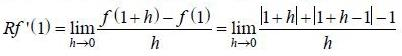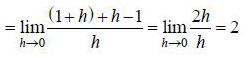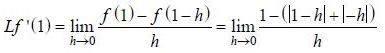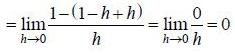Hence Lf'(1) ≠ Rf'(1)
∴ Derivative do not exist at x = 1.

*Multiple options can be correct
QUESTION: 13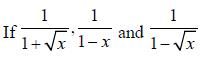are consecutive forms of a series, then series is

Solution:

Note that if a. b. c are three consecutive terms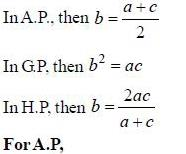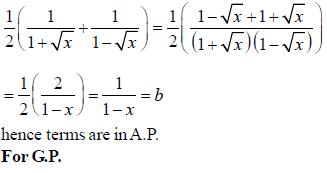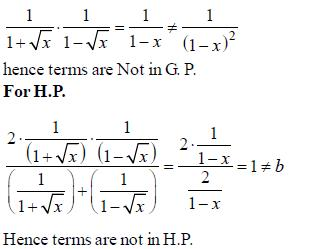*Multiple options can be correct
QUESTION: 14

The diffemetial equation representing the family of curves. y2 = 2c (x + √c). where c is positive parameter is of

Solution: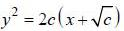......(1)
differentiating both side, we have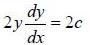i.e.c =yy’ ...(2)
from (1) we have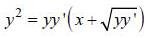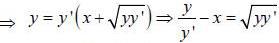Squaring both side we have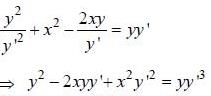Hence order of differential equation is 1 and degree is 3.

*Multiple options can be correct
QUESTION: 15

The equations of lines which pass through the point (3, -2) and are inclined at 60° to the line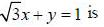Solution: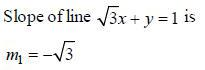Let slope of a line making 60° angle with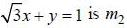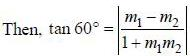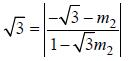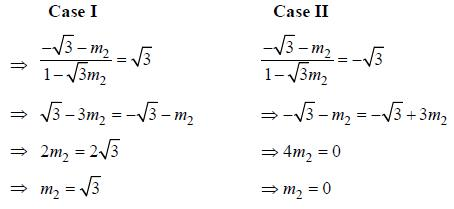So. there will be two lines of such type. One is having slope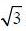and the otehr one is having slope 0.
Therefore.
Line 1: passing through (3, -2) and slope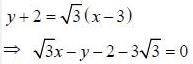Line 2: passing through (3.-2) and slope 0.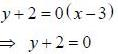Option (A) and (C) are correct.

*Answer can only contain numeric values
QUESTION: 16

The number of arrangements of the letters of the word BANANA in which the two N’s do not appear adjacently is _____________.

Solution:

In BANANA. Letter A reapets 3 times and N reapets 2 times.
Total number of arrangements of word BANANA is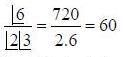Let both N s are appear together, then they are considered is single letter.
In this way total number of arrangements are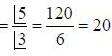Hence total number of arrangements where N do not appear adjacently is = 60 - 20 = 40

*Answer can only contain numeric values
QUESTION: 17

On the interval [0,1], the function f(x) = x25 (1 - x)75 takes its maximum value at the point _______.

Solution: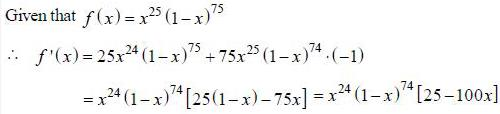For critical point, we have f'(x) = 0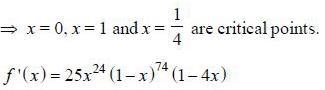Note that sign of f' (x) depends on the sign of (1 - 4x).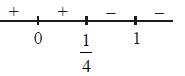hence f (x) is increasing when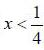and f (x) is decreasing when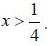∴ f (x) is maximum at x = 1/4

*Answer can only contain numeric values
QUESTION: 18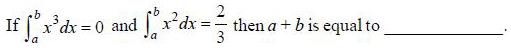Solution: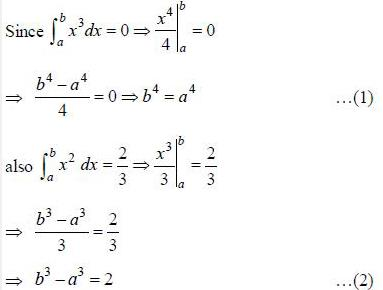Clearly from options, we have
b = 1. a = -1

*Answer can only contain numeric values
QUESTION: 19

If E = {1,2,3,4} and F = {1,2}, then the niunber of onto functions on E to F is ______.

Solution:

n(E) = 4     n(F) = 2
Then total number of onto functions from E to F are =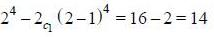Note: If n(A) = n and n(B) = m then total number of onto functions from A to B are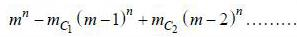*Answer can only contain numeric values
QUESTION: 20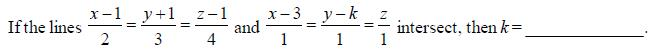Solution: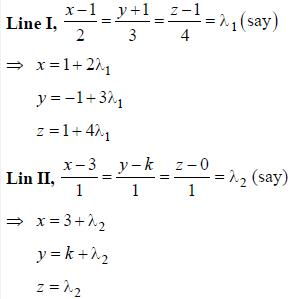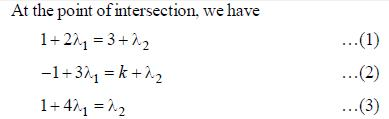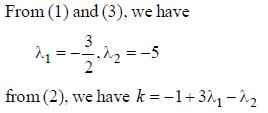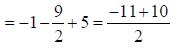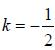*Answer can only contain numeric values
QUESTION: 21

The distance between the lines 3x + 4y = 9 and 6x + 8y = 15 is _______.

Solution:

3x + 4y = 9 and 6x + 8y = 15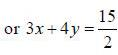We know that the distance between the two parallel lines ax + by = c1 and ax + by = c2 is